﻿ 一种SLF/ULF频段基于磁通负反馈的钻孔电磁波接收天线

# 一种SLF/ULF频段基于磁通负反馈的钻孔电磁波接收天线A Borehole Electromagnetic Wave Receiving Antenna Based on Flux Negative Feedback Technology in SLF/ULF Band

Abstract: At present, electromagnetic wave CT at home and abroad has electric antenna system based on electric dipole model and magnetic antenna system based on magnetic dipole model. Because the electric antenna needs to meet the antenna criterion (1/4 or 1/2 of the wavelength), the length is long. When the propagation distance of the electric antenna model is comparable to the scale, the strong nonlinearity and directivity of the near field will be affected by the structure, so the equiva-lence of the electric dipole model is poor. The electromagnetic wave of CT instrument based on magnetic antenna has good equivalence due to the characteristics of frequency structure. In a major national project, according to the actual requirements, the borehole electromagnetic wave CT sys-tem based on magnetic dipole model technology is adopted. The system requires the antenna to receive alternating magnetic field, the frequency band is 30 Hz~3000 Hz (SLF/ULF), and the sensi-tivity in the pass band should be flat. In this paper, the receiving antenna is theoretically analyzed and designed by using flux negative feedback technology combined with noise matching analysis, and the corresponding antenna prototype is made. After laboratory calibration and field test, each index of the antenna meets the project requirements.

1. 引言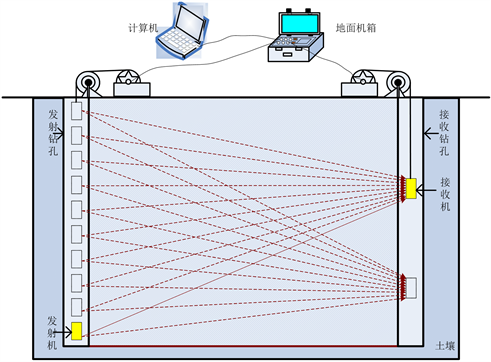Figure 1. Schematic diagram of cross hole electromagnetic wave tomography system (CT)

2. 工作原理

2.1. 接收天线组成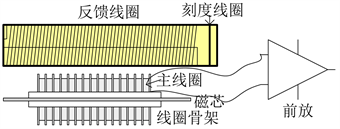Figure 2. Composition of receiving antenna

2.2. 接收天线模型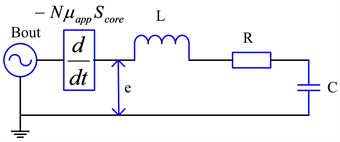Figure 3. Receiving coil model

$e=N\frac{\text{d}\varphi }{\text{d}t}=N\frac{\text{d}\left({B}_{core}{S}_{core}\right)}{\text{d}t}=N{\mu }_{app}\frac{\text{d}\left({B}_{out}{S}_{core}\right)}{\text{d}t}={\mu }_{app}N{S}_{core}\frac{\text{d}{B}_{out}}{\text{d}t}=jN{\mu }_{app}{S}_{core}{B}_{out}\omega$ (1)

${V}_{out}=e\left(j\omega \right)×\frac{1/jC\omega }{R+jL\omega +1/jC\omega }=\frac{j\omega {\mu }_{app}N{S}_{core}{B}_{out}}{\left(1-LC{\omega }^{2}\right)+j\omega RC}$ (2)

${T}_{VB0}\left(j\omega \right)=\frac{{V}_{out}}{{B}_{out}}=\frac{j\omega {\mu }_{app}N{S}_{core}}{\left(1-LC{\omega }^{2}\right)+j\omega RC}$ (3)

$N=40000$${S}_{core}=0.000314\text{\hspace{0.17em}}{\text{m}}^{2}$${\mu }_{app}=950$ 时，绘制其传递函数如图4所示：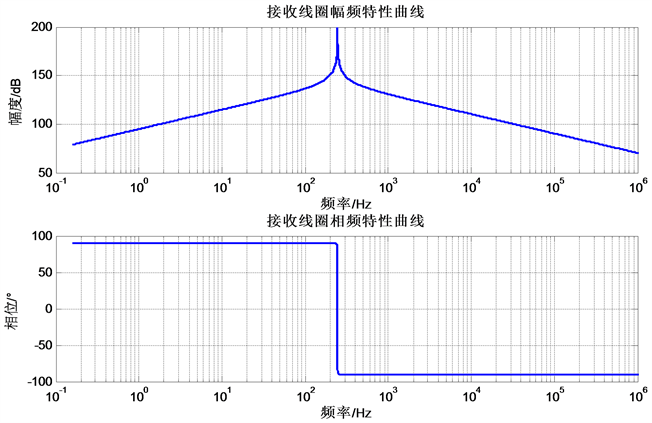Figure 4. Transfer function of receiving antenna without magnetic feedback

2.3. 磁通负反馈

${\varphi }_{1}={\mu }_{app}N{B}_{out}{S}_{core}+LI-{M}_{coil}{I}_{f}$ (4)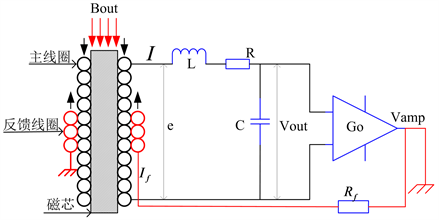Figure 5. Receiving antenna model with magnetic feedback

$e=\frac{\text{d}{\varphi }_{1}}{\text{d}t}\cong {\mu }_{app}N{S}_{core}\frac{\text{d}{B}_{out}}{\text{d}t}-\frac{\text{d}\left({M}_{coil}I\right)}{\text{d}t}={\mu }_{app}N{S}_{core}\frac{\text{d}{B}_{out}}{\text{d}t}-\frac{{G}_{o}{M}_{coil}}{{R}_{f}}\frac{\text{d}{V}_{out}}{\text{d}t}$ (5)

$e\left(j\omega \right)=j\omega \left({\mu }_{app}N{S}_{core}{B}_{out}-\frac{{G}_{o}{M}_{coil}}{{R}_{f}}{V}_{out}\right)$ (6)

${V}_{out}=e\left(j\omega \right)×\frac{1}{\left(1-LC{\omega }^{2}\right)+j\omega RC}=\frac{j\omega {\mu }_{app}N{S}_{core}{B}_{out}}{\left(1-LC{\omega }^{2}\right)+j\omega \left(RC+\frac{{G}_{o}M}{{R}_{f}}\right)}$ (7)

${T}_{VB1}\left(j\omega \right)=\frac{{V}_{amp}}{{B}_{out}}=\frac{{G}_{o}{V}_{out}}{{B}_{out}}=\frac{j\omega {\mu }_{app}{G}_{o}N{S}_{core}}{\left(1-LC{\omega }^{2}\right)+j\omega \left(RC+\frac{M}{{R}_{f}}{G}_{o}\right)}$ (8)

2.4. 磁芯的椭球体等效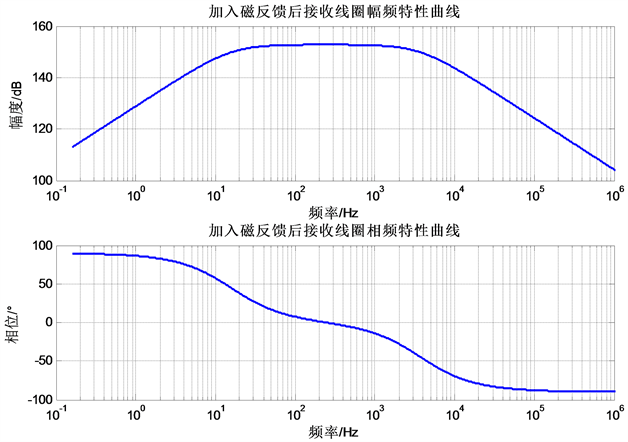Figure 6. Frequency characteristics of antenna after adding magnetic feedback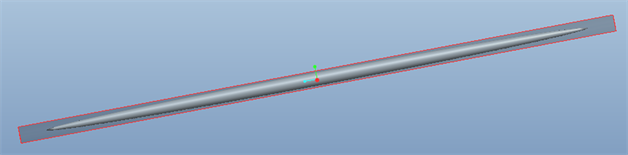Figure 7. The rod magnetic core is equivalent to an ellipsoid

${\mu }_{app}=\frac{{B}_{core}}{{B}_{out}}=\frac{{\mu }_{R}}{1+{N}_{Z}\left({\mu }_{R}-1\right)}=\frac{{\mu }_{R}}{1+\left(\frac{{\mu }_{R}-1}{{m}^{2}-1}\right)×\left[\frac{m}{\sqrt{{m}^{2}-1}}\mathrm{ln}\left(m+\sqrt{{m}^{2}-1}\right)-1\right]}$ (9)

2.5. 磁感应强度等效噪声NEMI

NEMI是Noise Equivalent Magnetic Induction (磁感应强度等效噪声)的简称，等效接收系统输入噪声水平，是接收系统测量微弱磁场能力的直接体现。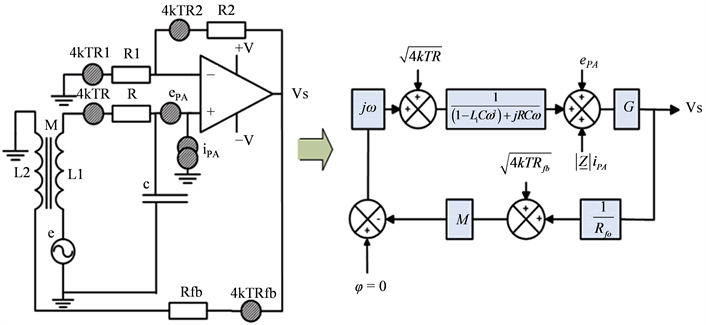Figure 8. Equivalent model of receiving noise

$NEMI\left(f\right)=\sqrt{\frac{PS{D}_{out}\left(f\right)}{T{\left(j\omega \right)}^{2}}}$ (10)

${T}_{VB1}\left(j\omega \right)=\frac{{V}_{amp}}{{B}_{out}}=\frac{{G}_{o}{V}_{out}}{{B}_{out}}=\frac{j\omega {\mu }_{app}{G}_{o}N{S}_{core}}{\left(1-LC{\omega }^{2}\right)+j\omega \left(RC+\frac{M}{{R}_{f}}{G}_{o}\right)}$ (11)

$PS{D}_{out}\left(f\right)=PS{D}_{R}+PS{D}_{{e}_{PA}}+PS{D}_{{i}_{PA}}+PS{D}_{{R}_{f}}$ (12)

$PS{D}_{R}=4kTR\frac{{G}^{2}}{{\left(1-LC{\omega }^{2}\right)}^{2}+{\left(RC\omega +\frac{GM\omega }{{R}_{fb}}\right)}^{2}}$ (13)

$PS{D}_{{e}_{PA}}\left({\text{V}}^{\text{2}}/\text{Hz}\right)={e}_{PA}^{2}\frac{{G}^{2}\left({\left(1-LC{\omega }^{2}\right)}^{2}+{\left(RC\omega \right)}^{2}\right)}{{\left(1-LC{\omega }^{2}\right)}^{2}+{\left(RC\omega +\frac{GM\omega }{{R}_{fb}}\right)}^{2}}$ (14)

$PS{D}_{{i}_{PA}}\left({\text{V}}^{\text{2}}/\text{Hz}\right)={i}_{PA}^{2}\frac{|Z|{G}^{2}\left({\left(1-LC{\omega }^{2}\right)}^{2}+{\left(RC\omega \right)}^{2}\right)}{{\left(1-LC{\omega }^{2}\right)}^{2}+{\left(RC\omega +\frac{GM\omega }{{R}_{fb}}\right)}^{2}}$ (15)

$|Z|$ 为传感器阻抗模值为：

$|Z|=\sqrt{\frac{{R}^{2}+{\left(L\omega \right)}^{2}}{{\left(1-LC{\omega }^{2}\right)}^{2}+{\left(RC\omega \right)}^{2}}}$ (16)

$PS{D}_{Rf}=4kT{R}_{f}$ (17)

2.6. 绝对灵敏度和电压灵敏度

${S}_{a}=\frac{e}{{B}_{out}\cdot f}=\frac{|e\left(j\omega \right)|}{{B}_{out}\cdot f}=2\pi N{\mu }_{app}{S}_{core}=\frac{2\pi N{S}_{core}{\mu }_{R}}{1+\left(\frac{{\mu }_{R}-1}{{m}^{2}-1}\right)×\left[\frac{m}{\sqrt{{m}^{2}-1}}\mathrm{ln}\left(m+\sqrt{{m}^{2}-1}\right)-1\right]}$ (18)

${\mu }_{R}=160000$，长径比 $m>10$ 时：

${S}_{a}=\frac{320000\pi N{S}_{core}}{1+\left(\frac{160000-1}{{m}^{2}-1}\right)×\left[\frac{m}{\sqrt{{m}^{2}-1}}\mathrm{ln}\left(m+\sqrt{{m}^{2}-1}\right)-1\right]}\cong \frac{2\pi N{S}_{core}{m}^{2}}{\mathrm{ln}\left(2m\right)-1}$ (19)

$|{T}_{VB1}\left(j\omega \right)|=\frac{\omega {\mu }_{app}{G}_{o}NS}{\sqrt{{\left(1-LC{\omega }^{2}\right)}^{2}+{\omega }^{2}{\left(RC+\frac{{G}_{o}M}{{R}_{f}}\right)}^{2}}}$ (20)

3. 天线设计

3.1. 天线材料选择

3.2. 天线屏蔽层设计

3.3. 天线参数设计

$L=\lambda \frac{{N}^{2}{\mu }_{0}{\mu }_{app}S}{l}=1680\text{\hspace{0.17em}}\text{H}$ (21)

$R=\rho N\frac{\left(d+N{\left({d}_{w}+t\right)}^{2}/{L}_{w}\right)}{{d}_{w}^{2}}=418\text{\hspace{0.17em}}\Omega$ (22)

$C=\frac{\pi {\epsilon }_{0}{\epsilon }_{r}{l}_{w}}{t\left({n}_{l}-1\right)}\left(d+2{n}_{l}\left({d}_{w}+t\right)\right)=0.3\text{\hspace{0.17em}}\text{nF}$ (23)

$M=\frac{L{N}_{f}}{N}\cong 4.2$ (24)

$|{T}_{VB1}\left(j\omega \right)|\cong \frac{{\mu }_{app}N{S}_{core}{R}_{f}}{M}$ (25)

$|{T}_{VB1}\left(j\omega \right)|=\frac{{\mu }_{app}N{S}_{core}{R}_{f}}{M}=2842.4{R}_{f}\ge 5×{10}^{8}\text{ }\text{ }\text{V}/\text{T}$ (26)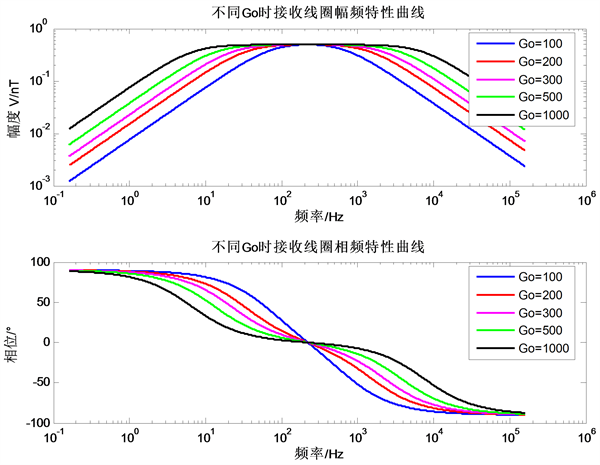Figure 9. Sensitivity curve of receiving antenna under different magnificationFigure 10. NEMI curve of receiving system

3.4. 前置放大器设计

3.4.1. 噪声匹配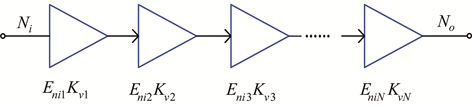Figure 11. Schematic diagram of cascaded amplifier

${E}_{ni}=\sqrt{{E}_{ni1}^{2}+\frac{{E}_{ni1}^{2}}{{K}_{v1}^{2}}+\frac{{E}_{ni2}^{2}}{{K}_{v1}^{2}{K}_{v2}^{2}}+\frac{{E}_{ni3}^{2}}{{K}_{v1}^{2}{K}_{v2}^{2}{K}_{v3}^{2}}+\cdots +\frac{{E}_{niN}^{2}}{{K}_{v1}^{2}{K}_{v2}^{2}{K}_{v3}^{2}\cdots {K}_{v\left(N-1\right)}^{2}}}$ (27)Figure 12. First stage amplifier circuit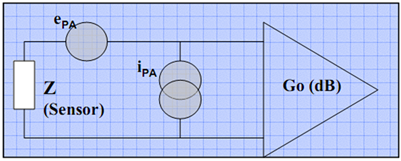Figure 13. Connection between the first stage amplifier and the receiving coil

${E}_{ni1}^{}=\sqrt{{e}_{PA}^{2}+{\left(|Z|{i}_{PA}\right)}^{2}}=\sqrt{{e}_{PA}^{2}+\frac{\left({R}^{2}+{\left(L\omega \right)}^{2}\right){i}_{PA}^{2}}{{\left(1-LC{\omega }^{2}\right)}^{2}+{\left(RC\omega \right)}^{2}}}$ (28)

3.4.2. 次级放大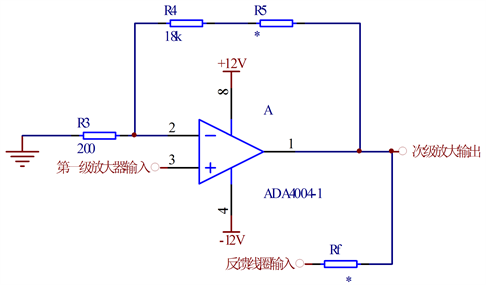Figure 14. Second stage amplifier circuit

4. 天线制造及测试Table 1. Parameters of receiving antenna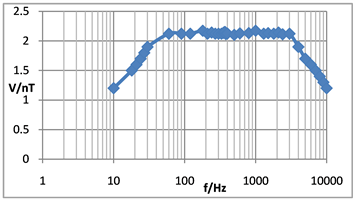Figure 15. Antenna scale and sensitivity characteristic diagram

5. 结论

 Alumbaugh, D., DePavia, L., 等. 油气藏井间监测新技术及其应用[J]. 油田新技术, 2009, 21(2): 38-47.

 巨汉基, 朱万华, 方广有. 磁芯感应线圈传感器综述[J]. 地球物理学进展, 2010, 25(5): 1870-1876.

 Baltag, O., Costandache, D., Rau, M., Iftemie, A. and Rau, I. (2010) Dynamic Shielding of the Magnetic Fields. Advances in Elec-trical and Computer Engineering, 10, 135-142.
https://doi.org/10.4316/aece.2010.04022

 张平, 史美光. 标定感应式磁力仪探头绝对灵敏度的新方法[J]. 地球物理学报, 1984, 27(5): 463-470.

 高晋占. 微弱信号检测[M]. 北京: 清华大学出版社, 2004: 260-272.

 朱万华, 闫彬, 等. 用于MT方法的超小型感应式磁场传感器[J]. 地球物理学进展, 2015, 30(2): 894-898.

Top